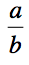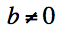Example Questions

Example Question #1 : How To Find The Amount Of Rational Numbers Between Two Numbers

How many rational numbers are there between 0 and 5?

Infinitely many

0

5

6

Infinitely many

Explanation:

Rational numbers are written in the formwhere; therefore, we can write infinitely many combinations of rational numbers between 0 and 1, e.g. 1/2, 1/3, 1/4, 1/5, 1/6 . . . .

Example Question #2 : How To Find The Amount Of Rational Numbers Between Two Numbers

A flight from Boston to Los Angeles lasts about 6 hours. The time zone difference is such that it is 3 hours later in Boston. If the flight leaves Boston at 8:00 AM, what will be the local time in Los Angeles when the plane arrives?

11:00 AM

3:00 PM

2:00 PM

1:00 PM

5:00 PM

11:00 AM

Explanation:

The plane will land in Los Angeles at 2:00 PM Boston time (8:00 AM + 6 hours = 2:00 PM).

Going west, subtract the time zone difference. Going east, add the time zone difference.

So, 2:00 PM Boston time becomes 11:00 AM local time in Los Angeles (2:00 PM – 3 hours = 11:00 AM).

Example Question #3 : How To Find The Amount Of Rational Numbers Between Two Numbers

How many rational numbers are between 1 and 2?

None

Infinitely many

Just one

Two: 1 and 2